## Dynamics Exam2 and Problem Solutions

Dynamics Exam2 and  Problem Solutions

1. Position time graphof the box is given below. Find the friction constant between box and surface? (g=10m/s2)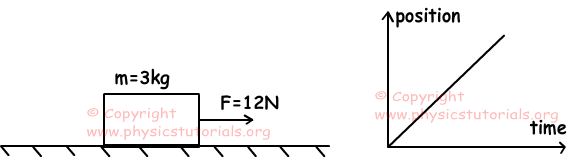Slope of the graph gives us velocity of the box. Since the slope of the position time graph is constant, velocity of the box is also constant. As a result, acceleration of the box becomes zero.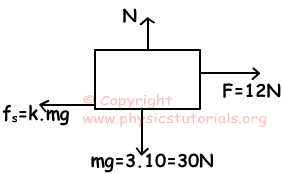Fnet=F-fs=m.a=0

Fnet=fs

fs=12

k.mg=12

k.3.10=12

k=0,4

2. If the acceleration of the system given below is 3m/s2, find the friction constant between box and surface. (sin370=0,6, cos370=0,8, sin450=cos450=√2/2)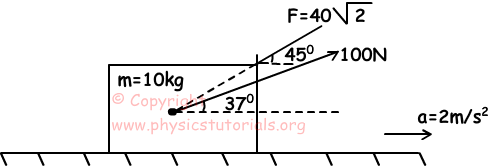Free body diagrams of the system are given below.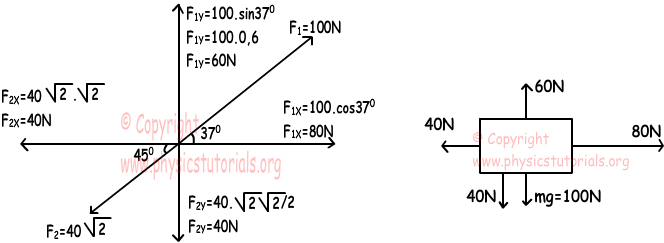Acceleration of the 10 kg box is 2m/s2. Thus, net force acting on this box is;

Fnet=m.a

Fnet=10.2=20N

Normal force of the box is;

N=100+40-60=80N

Fnet=80-40-Ffriction

20=80-40-k.80

k.80=20

k=1/4

3. Net force vs. time graph of object is given below. If displacement of this object between t-2t is 75m, find the displacement of the object between 0-3t.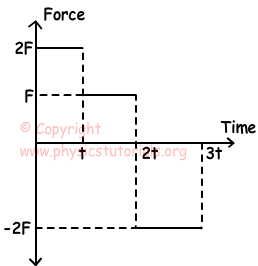We draw acceleration vs. time graph using force vs time graph of the object.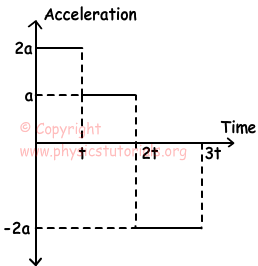Area under the graph gives velocity.

If we say at=V then,

Vt=2V

V2t=3V

V3t=V

We draw velocity vs. time graph now.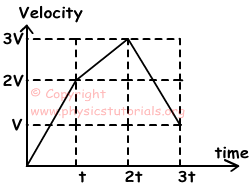Area under the velocity vs. time graph gives us displacement of the object.

0-t: ΔX1=2Vt/2=Vt

t-2t: ΔX2=5/2.Vt

2t-3t: ΔX3= 2.Vt

We know ΔX2=5/2.Vt=75m, Vt=30m

Total displacement=ΔX1+ΔX2+ΔX3=Vt+5/2.Vt+2Vt

Total displacement=30+75+2.30=165m

4. An object is pulled by constant force F from point A to C. Draw the acceleration vs. time graph of this motion. (F>mg.sinθ and surface is frictionless.)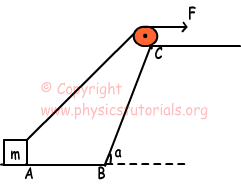Motion of the box between points A to B: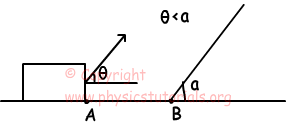F.cosθ=m.a1

When the object gets closer to point B, θ becomes larger, and value of cosθ decreases. Thus, a1 decreases between the points A -B.

Motion between points B-C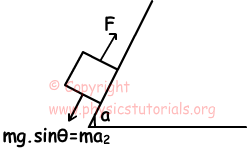Net force between points B and C is constant. Thus, a2 is also constant. Acceleration vs. time graph of the box is given below;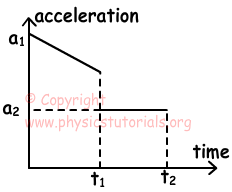5. System in the given picture below, box moves under the effect of applied force and gravity with 1m/s2 acceleration. Find the friction constant between the box and surface.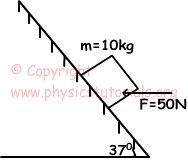Free body diagram of the system is given below;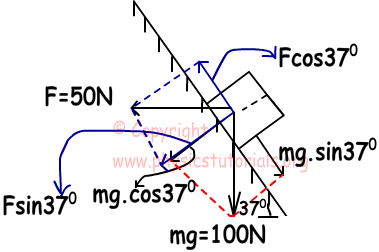Forces acting on the box perpendicularly;

30+80=110N

Box moves downward with 1m/s2 acceleration.

Fnet=m.a

60-40-Ffriction=10.1

20-k.110=10

10=110k

k=1/11

Author: